# GSEB Solutions Class 12 Chemistry Chapter 3 Electrochemistry

Gujarat Board GSEB Textbook Solutions Class 12 Chemistry Chapter 3 Electrochemistry Textbook Questions and Answers, Additional Important Questions, Notes Pdf.

## Gujarat Board Textbook Solutions Class 12 Chemistry Chapter 3 Electrochemistry

### GSEB Class 12 Chemistry Electrochemistry InText Questions and Answers

Question 1.
How would you determine the standard electrode potential of the system Mg2+| Mg?
$$\mathrm{E}_{\mathrm{Mg}^{2+} / \mathrm{Mg}}^{\circ}$$ can be determined by forming a cell with standard hydrogen electrode (SHE).
Mg(s) | Mg2+ 1 M || H+g | H2 (1 atm) Pt.Question 2.
Can you store copper sulphate solutions in a zinc pot?
The electrode potential $$\mathrm{E}_{\mathrm{Cu}^{2}+\mathrm{Cu}}^{\circ}$$ = +0.34 V and $$\mathrm{E}_{\mathrm{Zn}^{2}+\mathrm{Zn}}^{\circ}$$ = – 0.76 V. Since the electrode potential of Cu is higher than that of Zn, copper sulphate cannot be stored in zinc pot.

Question 3.
Consult the table of standard electrode potentials and suggest three substances that can oxidize ferrous ions under suitable conditions?
Only those species can oxidise ferrous ions (Fe2+) whose standard reduction potential is more positive than 0.77V. Thus, suitable oxidising agents will be F2, Cl2, Br2.

Question 4.
Calculate the potential of the hydrogen electrode in contact with a solution whose pH is 10.
H+ + 1e → $$\frac{1}{2}$$H2
E = E° – $$\frac{0.0591}{n}$$ log $$\frac{1}{\left[\mathrm{H}^{+}\right]}$$
= E° – $$\frac{0.0591}{1}$$ PH = 0 – 0.591 × 10 = – 0.591 VQuestion 5.
Calculate the emf of the cell in which the following reaction takes place
Ni(s) + 2Ag+(0.002 M) → Ni2+(0.160 M)) + 2Ag(s)
Given that E°(cell) = 1.05 V
E = E° – $$\frac{0.0591}{n}$$ log $$\frac{\left[\mathrm{Ni}^{2+}\right]}{\left[\mathrm{Ag}^{+}\right]^{2}}$$ = 1.05 – $$\frac{0.0591}{2}$$ log $$\frac{(0.16)}{(0.002)^{2}}$$
E = 0.915 V

Question 6.
The cell in which the following reaction occurs:
2Fe3+(aq) + 2I(aq) → 2Fe2+(aq) + I(2s) has E°(cell) = 0.236 V at 298 K. Calculate the standard Gibbs energy and the equilibrium constant of the cell reaction?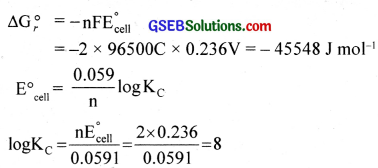KC = antilog (8) = 108Question 7.
Why does the conductivity of a solution decrease with dilution?
Because number of ions per cm3 decreases.

Question 8.
Suggest a way to determine the $$\Lambda_{\mathrm{m}}^{0}$$ value of water?
$$\wedge_{\mathrm{mH}_{2} \mathrm{O}}^{0}=\wedge_{\mathrm{m} \mathrm{NaOH}}^{0}+\wedge_{\mathrm{mHCl}}^{0}-\wedge_{\mathrm{m} \mathrm{NaCl}}^{0}$$

Question 9.
The molar conductivity of 0.025 mol L-1 methanoic acid is 46.1 S cm2mol-1. Calculate its degree of dissociation and dissociation constant. Given $$\lambda_{\left(\mathrm{H}^{+}\right)}^{0}$$ = 349.6 S cm2 mol-1 and $$\lambda_{\left(\mathrm{HCOO}^{-}\right)}^{0}$$ = 54.6 S cm2mol-1
$$\wedge^{0} \mathrm{~m}(\mathrm{HCOOH})=\lambda_{\mathrm{H}+}^{0}+\lambda_{\mathrm{HCOO}^{-}}^{-}$$
= 349.6 + 54.6 = 404.2 S cm2mol-1
Degree of dissociation, α = $$\frac{\lambda_{\mathrm{m}}^{\mathrm{c}}}{\lambda_{\mathrm{m}}^{0}}$$ = $$\frac{46.1}{404.2}$$ = 0.114
Ka = Cα2 = 0.025 × (0.114)2 = 3.249 × 10-4Question 10.
If a current of 0.5 ampere flows through a metallic wire for 2 hours, then how many electrons would flow through the wire?
Q = It = ne
0.5 × 2 × 60 × 60s = n × 1.6 × 10-19
n = $$\frac{0.5 \times 2 \times 60 \times 60}{1.6 \times 10^{-19}}$$ = 2.25 × 1022

Question 11.
Suggest a list of metals that are extracted electrolytically?
The metals like Na, K, Mg, Al, Ca etc. i.e., reactive metals can be extracted electrolytically.

Question 12.
Consider the reaction: Cr2O7 + 14H+ + 6e → 2Cr3+ + 7H2O. What is the quantity of electricity in coulombs needed to reduce 1 mol of Cr2O2-7?
Reduction of 1 mol of Cr2O7 requires 6 mol of electricity.
= 6 × 6.02 × 1023 × 1 .6 × 10-19C = 577920 C

Question 13.
Write the chemistry of recharging the lead storage battery, highlighting all the materials that are involved during recharging. During this process, lead is deposited at the cathode, lead oxide is formed at the anode and H2SO4 is generated in the cell?
2PbSO4 + 2H2O → Pb(s) + PbO2(s) + 2H2SO4

Question 14.
Suggest two materials other than hydrogen that can be used as fuels in fuel cells?
Methanol (CH3OH), propane (C3H8)Question 15.
Explain how rusting of iron is envisaged as setting up of an electrochemical cell?
Corrosion is a process which involves the slow destruction of a metal as a result of its reaction with moisture and other gases present in the atmosphere. Corrosion always occurs at anodic areas. More reactive metals are corroded more easily.

Corrosion is enhanced by the presence of (a) impurities (Pure iron does not undergo rusting), (b) air and moisture, (c) electrolytes and (d) strains in metals like dents, scratches etc. Corrosion of iron is called rusting. Rust is a hydrated form of ferric oxide. Fe2O3 x H2O. Tarnishing of silver, formation of green coating on copper and bronze etc. are other examples of corrosion.

Mechanism of corrosion (Rusting):
During rusting impure surface of iron behaves like an electrochemical cell in presence of water known as corrosion cell. In corrosion cell, pure iron acts as anode and impure iron acts as cathode.

The iron tends to oxidise according to the half-reaction,
Fe(s) → Fe2+(aq) + 2e, $$\mathrm{E}_{\mathrm{Fe}^{2} / \mathrm{Fe}}^{\mathrm{o}}$$ = -0.44 V ………………. (1)
This spot behaves as anode. At the same time, oxygen molecules in the solution are reduced.
O2 + 4H+ + 4e → 2H2O, $$\mathrm{E}_{\mathrm{H}^{+}\left|\mathrm{O}_{2}\right| \mathrm{H}_{2} \mathrm{O}}^{\circ}$$ = 1.23 V ………………. (2)

(It is believed that W ions are available from H2CO3 formed due to dissolution of CO2 in water). This spot behaves as a cathode.

Adding these two half cell reactions, (after multiplying eqn (1) by 2)
2Fe + O2 + 4H+ → 2Fe2+ + 2H2O ……………….. (3)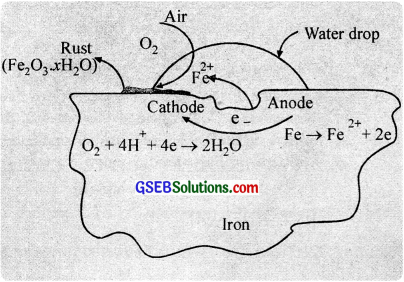The ferrous ions are further oxidised by at atmospheric oxygen to form rust. Fe2O3.xH2O.
4Fe2+ + O2 + 4H2O → 2Fe2O3 + 8H+ ……………………. (4)
Fe2O3 + xH2O → Fe2O3.xH2O(s) (Rust) …………………. (5)

The two half reactions (1) and (2) are taking place at different locations, which suggest that corrosion occurs by an electrochemical mechanism. The iron surface may be thought of as consisting of a series of tiny voltaic cells. At anodic centres, iron is oxidised to Fe2+ ions and at cathodic centres, oxygen is reduced.

Electrons are transferred through the iron, which acts like an external circuit. The electrical circuit is completed by the flow of ions through the water solution or the film covering of iron. Very pure iron does not rust easily. But the presence of acids, salts, electrolytes, air, moisture etc accelerate the rate of rusting. Thus, (i) a perfectly dry metal surface is not attacked by oxygen (because of incomplete circuit) and (ii) corrosion occurs more readily in sea water than ¡n fresh water (sea water supplies the necessary ions for conduction).

Prevention of corrosion:

(i) By coating with a suitable material (Barrier protection):
In this method, rusting is prevented by making a barrier between the metal and the atmosphere. This is usually done by coating the surface with paints, grease, metals like Zn, Cu, Sn, Ni etc.. adherent film of iron phosphate, chemicals like bisphenol etc.

(ii) Sacrificial protection:
This method involves the coating of the surface of iron with a layer of metal which is more active than iron (e.g. Zn, Cr, Ni etc.) and this prevents the loss of electrons from iron. The active metal loses electrons in preference to iron and goes into ionic state.

With the passage of time, the covering metal gets consumed. But as long as it ¡s present on the surface of iron, it will protect the iron from rusting and does not allow even the nearly exposed surface of iron to corrode. Zinc is commonly used to coat iron surfaces. The process of coating a thin film of zinc on iron is known as galvanisation.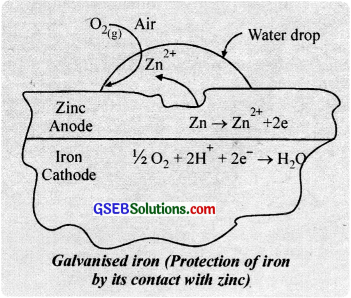Zinc ($$E_{z n^{2+} / Z n}^{0}$$ = -0.76 V) is more electropositive than iron ($$E_{Fe^{2+} / Fe}^{0}$$ = – 0.44 V), so zinc possesses greater tendency to lose electrons than iron. Thus, zinc protects iron from rusting at its own cost. Consequently. if by reason, the protective layer of zinc is scratched, atmospheric air and moisture dissolve zinc and not iron. Thus, zinc acts as the anodic region; while iron as the cathodic region.
Zn → Zn2+ + 2e (anodic region)
H2O → H+ + OH
2H+(Fe) + 2e → H2 + Fe (cathodic region)

(iii) By cathodic protection:
In cathodic protection, iron is made as the cathode halfcell so that it will not lose electrons and dissolve. This can be achieved by attaching a more active metal such as Mg or Zn to the iron. Here the active metal acts as anode by losing electrons and iron becomes cathode.
(Mg → Mg2+ + 2$$\bar{e}$$)

(iv) Anti – rust solutions:
When a solution of alkaline phosphate or alkaline chromate is applied on iron objects, they form an insoluble, heat resistant iron phosphate or iron chromate coating and thus prevents rusting. Further the alkalinity of the solution removes the H+ ions which in turn decrease
rusting. Such solutions are used in car radiators.

### GSEB Class 12 Chemistry Electrochemistry Text Book Questions and Answers

Question 1.
Arrange the following metals in the order in which they displace each other from the solution of their salts?
Al, Cu, Fe, Mg and Zn.
Mg, Al, Zn, Fe, CuQuestion 2.
Given the standard electrode potentials, K+/K = -2.93 V, Ag+/Ag = 0.80V, Hg2+/Hg = 0.79 V Mg2+/Mg = -2.37 V, Cr3+/Cr = – 0.74V. Arrange these metals in their increasing order of reducing power?
The reducing power of metals increases in the order Ag < Hg < Cr < Mg < K

Question 3.
Depict the galvanic cell in which the reaction Zn(s) + 2Ag+(aq) → Zn2+(aq) + 2e takes place. Further show.

1. which of the electrode is negatively charged?
2. the carriers of the current in the cell?
3. individual reaction at each electrode?

The cell can be represented as Zn(s)| Zn2+(aq)||Ag+(aq)|Ag(s)

1. Zinc electrode (anode) will be negatively charged.
2. The current will flow from silver electrode to zinc electrode.
• At anode: Zn(s) → Zn2+(aq) + 2e
• At cathode: Ag+(aq) + 1e → Ag

Question 4.
Calculate the standard cell potentials of galvanic cell in which the following reactions take place:
(i) 2Cr(s) + 3Cd2+(aq) → 2Cr3+(aq) + 3Cd
(ii) Fe2+ + Ag+(aq) → Fe3+(aq) + Ag(s)
Calculate the ∆rG° and equilibrium constant of the reactions.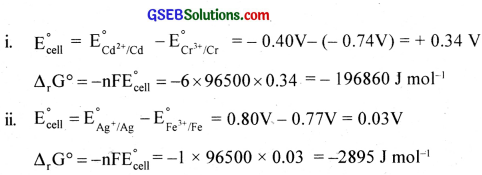Question 5.
Write the Nernst equation and emf of the following cells at 298 K: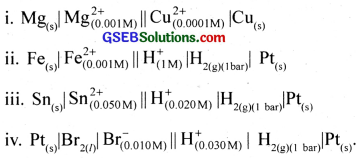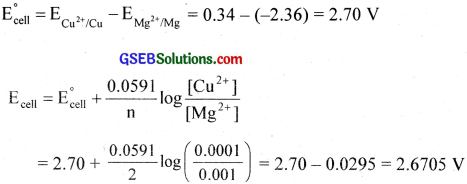Similarity

• Ecell = 0.53 V
• Ecell = 0.08 V
• Ecell = -1.052 V

Question 6.
In the button cells widely used in watches and other devices the following reaction takes place:
Zn(s) + Ag2O(s) + H2O(l) → Zn2+(aq) + 2Ag(s) + 2OH(aq) Determine ∆rG° and E° for the reaction?
Zn is oxidised and Ag2O is reduced.
$$\mathrm{E}_{\mathrm{cell}}^{\circ}=\mathrm{E}_{\mathrm{Ag}^{+} / \mathrm{Ag}}^{\circ}-\mathrm{E}_{\mathrm{Zn}^{2 *} / \mathrm{Zn}}^{\circ}$$ = 0.344 – (-0.76) = 1.104 V
$$\Delta_{r} \mathrm{G}^{0}=-\mathrm{nFE}_{\text {celi }}^{\circ}$$ = -2 × 96500 × 1.104 = -2.13 × 105J

Question 7.
Define conductivity and molar conductivity for the solution of an electrolyte. Discuss their variation with concentration?
(a) Variation of conductivity, k:
Conductivity is the conductance of 1 cm3 of the solution. Therefore, with decrease in concentration or increase in dilution, the number of ions present per unit volume of the solution decreases. Thus, the conductivit of the solution decreases. Thus, the conductivity of the solution decreases with decrease in concentration.

(b) Variation of molar conductivity, λm:
The molar conductance of electroly tes increases with decrease in concentration or increase in dilution. Howcvcr. the variation is different for strong electrolytes (eg. HCl, KCl, NaOH) and weak electrolytes (eg. CH3COOH. NH4OH)

(i) Strong electrolytes:
For strong electrolytes, the molar conductivity increases with decrease in concentration and reaches a maximum limiting value when the concentration approaches zero or at infinite dilution. This is known as $$\lambda_{\mathrm{m}}^{\infty}$$ or $$\lambda_{\mathrm{m}}^{0}$$.
The λm values with $$\sqrt{C}$$ for strong electrolytes HCl and KCl are shown in the graph.

The graph is a straight line at low concentration and can be extrapolated to zero concentration to get $$\lambda_{\mathrm{m}}^{0}$$. The variation can be represented by the equation
λm = $$\lambda_{\mathrm{m}}^{0}$$ – b$$\sqrt{C}$$ where the intercept is equal to $$\lambda_{\mathrm{m}}^{0}$$ and slope equal to – b. This equation is known as Dehye Huckel Onsager equation.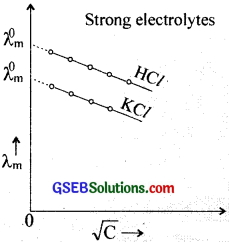Strong electrolytes are almost completely ionised even at moderate concentration. The molar conductance of such a solution in creases on further dilution due to the increase in the mobility speed of the ions since other interacting forces are minimised on dilution.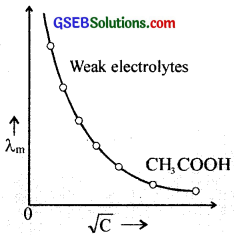(ii) Weak electrolytes:
For weak electrolytes, the value of λm will be low as compared lo those of strong electrolytes because they undergo partial ionisation only. Also, the graph ¡s not linear and $$\lambda_{\mathrm{m}}^{0}$$ cannot be determined graphically.

For weak electrolytes the degree of ionisation is very small in their concentrated solutions. On dilution, the degree of ionisation increases and hence the number of ions present in the solution increases and the molar conductance increases. Thus $$\lambda_{\mathrm{m}}^{0}$$ values of weak electrolytes are determined only by using Kohlrausch’s law.Question 8.
The conductivity of 0.20 M solution of KCl at 298 K is 0.0248 S cm-1. Calculate its molar conductivity?
$$\Lambda_{\mathrm{m}}$$ = $$\frac{1000k}{M}$$ = $$\frac{0.0248×1000}{0.2}$$ = 124 Scm2mol-1

Question 9.
The resistance of a conductivity cell containing 0.001M KCl solution at 298 K is 1500 Ω. What is the cell constant if conductivity of 0.001 M KCl solution at 298 K is 0.146 × 10-3 S cm-1?
Cell constant = Conductivity × Resistance
= 0.146 × 10-3 Scm-1 × 1500 Ω = 0.219 cm-1

Question 10.
Conductivity of 0.00241 M acetic acid is 7.896 × 10-5 S cm-1. Calculate its molar conductivity. If $$\Lambda_{\mathrm{m}}$$ for acetic acid is 390.5 S cm2 mol-1, what is its dissociation constant?
K = 7.896 × 10-5S cm-1
M = 0.00241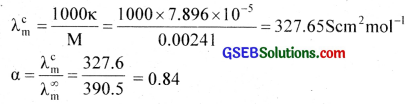Ka = Cα2 = 0.00241 × (0.84)2 = 1.85 × 10-5Question 11.
How much charge is required for the following reductions?

1. 1 mol of Al3+ to Al
2. 1 mol of Cu2+ to Cu
3. 1 mol of MnO4 to Mn2+

1. Al3+ + 3e → Al
Charge required = 3F

2. Cu2+ + 2e → Cu
Charge required = 2F

3. MnO4 + 8H+ + 5e → Mn2+ + H2O
Charge required = 5F

Question 12.
How much electricity in terms of Faraday is required to produce

1. 20.0 g of Ca from molten CaCl2
2. 40.0 g of Al from molten Al2O3

1. Ca2+ + 2e → Ca
2F can produce 1 mole (= 40 g) Ca
∴ To produce 20 g Ca requires, $$\frac{2F×20}{40}$$ = 1F

2. Al3+ + 3e → Al
3F can produce 1 mole (= 27 g) Al.
∴ To produce 40 g Al requires $$\frac{3F×40}{27}$$ = 4.44 F

Question 13.
How much electricity is required in coulomb for the oxidation of

1. 1 mol of H2O to O2
2. 1 mol of FeO to Fe2O3.

1. 2H2O → O2 + 4H+ + 4e
2mole – 4F
lmole – 2F
Electricity required for one mole H2O = 2F = 2 × 96500 coulomb.

2. 4FeO + O2 → 2Fe2O3
or Fe2+ → Fe3+ + e
1 mole – IF
1 × 96500 coulomb.Question 14.
A solution of Ni(NO3)2 is electrolysed between platinum electrodes using a current of 5 amperes for 20 minutes. What mass of Ni is deposited at the cathode?
Ni2+ + 2e → Ni
2F (2 × 96500 C) can produce 58.7 g of Ni,
Q = It = 5 × 20 × 60 = 6000 C
∴ 6000 C can deposit $$\frac{58.7×6000}{2×96500}$$ = 1.83 g

Question 15.
Three electrolytic cells A, B, C containing solutions of ZnSO4, AgNO3 and CuSO4, respectively are connected in series. A steady current of 1.5 amperes was passed through them until 1.45 g of silver deposited at the cathode of cell B. How long did the current flow? What mass of copper and zinc were deposited?
ZAg = 108
ZZn = 65.4
ZCu = 63.5
Reactions involved are
Zn2+ + 2e → Zn
Ag+ + 1e → Ag
Cu2+ + 2e → Cu
108 g of Ag is deposited by 1F = 96500 C
∴ 1.45 g Ag is deposited by $$\frac{96500}{108}$$ × 1.45 = 1297 C
Q = 1t
t = $$\frac{Q}{1}$$ = $$\frac{1297}{1.5}$$ = 864 s = 14 min 24 sec.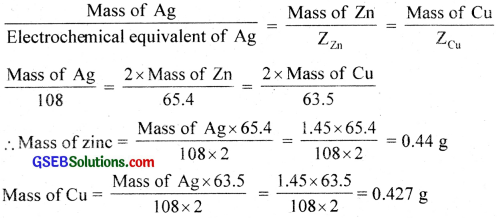Question 16.
Using the standard electrode potentials predict if the reaction between the following is feasible?

1. Fe3+(aq) and I(aq)
2. Ag+(aq) and Cu(s)
3. Fe3+(aq) and Br(aq)
4. Ag(s) and Fe3+(aq)
5. Br2(aq) and Fe2+(aq)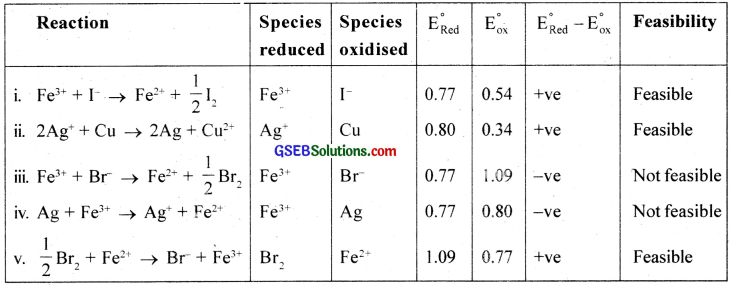Question 17.
Predict the products of electrolysis in each of the following:

1. An aqueous solution of AgNO3 with silver electrodes.
2. An aqueous solution of AgNO3 with platinum electrodes.
3. A dilute solution of H2SO4 with platinum electrodes.
4. An aqueous solution of CuCl2 with platinum electrodes.

1. AgNO3 → Ag+ + NO3
H2O ⇄ H+ + OH

At cathode:
Since Ag+ ions have lower potential than H+ ions, Ag+ ions will be deposited as Ag.

At anode:
NO3 ions attack Ag anode and hence Ag will dissolve in the solution.
Ag(s) → Ag+(aq) + e

2. At cathode: Same as above
At anode: Anode is not attackable and hence OH ions have lower discharge potential than NO3 ions and OH ions react to give O2.
OH → OH + e
40H → 2H2O + O2(g)

3. H2SO4 → 2H+ + SO2-4
H2O ⇄ H+ + OH
At cathode: 2H+ + 2e → H2
At anode: 4OH → 2H2O + O2 + 4e
i.e; H2 will be liberated at cathode and O2 at anode.

4. CuCl2 → Cu2+ + 2Cl
H2O ⇄ H+ + OH
At cathode: Cu2+ ions will be reduced in preference to H+ ions.
Cu2+ + 2e → Cu
At anode: Cl ions will be oxidised in preference to OH ions.
2Cl → Cl2 + 2e
i. e., Cu will be deposited on the cathode and Cl2 will be liberated at the anode.

Question 1.
Define molar conductivity of a solution.
The molar conductivity of a solution at a given concentration is the conductance of volume ‘V’ of a solution containing 1 mole of _ the electrolytic kept between two electrodes with cross-sectional area ‘A’ and distance of unit length.
Or,
Δm = $$\frac{\Delta}{1}$$ K
Now,
1 = 1 and Δ = V (volume containing 1 mole of the electrolyte)
∴ Δm = KVQuestion 2.
A galvanic cell is constructed out of Zn2+ | Zn and Ag+ | Ag half cells. Given?
$$\mathrm{E}^{\circ} \mathrm{zn}^{2}+\mathrm{Zn}$$ = -0.76 V.
$$\mathrm{E}^{\circ} \mathrm{Ag}^{+} / \mathrm{Ag}$$ = 0.80 V. Answer the following.

1. What is the cell reaction?
2. What is the e. m. f of the cell?
3. What are the signs of the two electrodes in the galvanic cell?
4. Which ion is the powerful oxidising agent?
5. Which metal is the most powerful reducing agent?

1. The cell reaction is
Zn + 2 Ag+ → Zn2+ + 2Ag
Zn → Zn2+ + 2e (oxidation half cell)
2Ag+ + 2e → 2Ag (reduction half cell)

2. e.m.f of the cell
$$\mathrm{E}_{\text {cell }}^{\circ}=\mathrm{E}_{\text {red(cathode) }}^{\circ}-\mathrm{E}_{\text {oxi(anode) }}^{\circ}=\mathrm{E}_{\mathrm{R}}^{\circ}-\mathrm{E}_{\mathrm{L}}^{\circ}$$ = +0.80 – (-0.76) = +1.56V

3. Anode is the negative terminal and cathode is the positive terminal in the galvanic cell.

4. The powerful oxidising agent is Ag+.

5. The most powerful reducing agent is Zn.Question 3.
Ramu found that the cell which he had used in torches are not rechargeable while that used in car are chargeable?

1. What are the basic differences between them?
2. Explain the working of batteries used in torches?
3. A dry cell becomes dead after a long time even if it has not been used. Give reason?
4. Which battery is used in digital watches? What are the advantages of them?

1. The cell which is used in torches is dry cell. It is an example of a primary cell In primary cells the electrode reactions cannot be reversed by external energy source. Therefore these are not rechargeable. While the battery used in a car is usually a lead storage cell and in these cells the reactions can be reversed by passing electric current through them. These are known as secondary cells and are rechargeable.

2. Dry cell consists of a zinc container containing a central graphite rod surrounded by powdered manganese dioxide (MnO2) and carbon. The space between the electrodes are filled with a paste of ZnCl2, and NH4Cl. Zinc container acts as the anode and graphite acts as the cathode.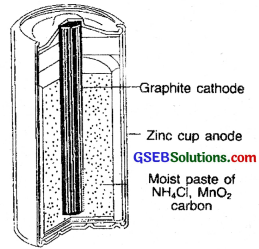The cell reactions are:
At anode: Zn(s) → Zn2+ + 2e
At cathode: 2MnO2(s) + 2NH+4 + 2e → Mn2O3(s) + 2NH3(g) + H2O
The e.m.f. of the cell is about 1.45 V.

3. A dry cell becomes dead after a long time because the acidic NH4Cl corrodes the zinc container.

4. Mercury cells are usually used in digital watches. The e.m.f of the cell is 1.35 V throughout its life. This is because, the overall cell reaction does not involve any ion in solution whose concentration can change.Question 4.
A car battery is made of lead dipping into sulphuric acid (Lead Accumulator). It can be recharged?

1. It is said to be a secondary cell. Do you agree? Substantiate your answer.
2. When the battery is used, a reaction takes place. Identify the change to this reaction when the battery is recharged and represent the reactions at the anode of the ceil
3. Is there any change in the concentration of H2SO4 during discharging and recharging the cell? Explain.

1. Yes. A cell that can be used again and again by recharging is said to be a secondary cell.

2. The reaction is reversed.
At the anode during discharging oxidation takes place
Pb(s) → Pb2+ + 2e
During recharging, reduction takes place
Pb2+ + 2e → Pb(s)

3. During discharging, the concentration of H2SO4 decreases, and during recharging it increases. During discharging process, Pb has converted to PbSO4 thereby concentration of H2SO4 decreases.
Pb + PbO2 + 2H2SO4 → 2PbSO4 + 2 H2O

During recharging PbSO4 reduces to give SO2-4 ions and the concentration of H2SO4 increases
2 PbSO4 + 2 H2O → Pb + PbO2+ 2H2SO4.

Question 5.
In Appollo space programme the fuel cell are used not only for electric power but also to prepare H2O for drinking?

1. What do you understand by a fuel cell?
2. Explain the working of an H2 – O2 fuel cell?
3. What are the advantages of fuel cells over ordinary cells?

1. A fuel cell is a device that can convert a portion of free energy change into electrical energy. The fuel used is H2, CH4, CO, etc. The common fuel cell is H2 – O2, fuel cell.

2. The H2 – O2 fuel cell consists of a porous carbon electrode containing catalysts like platinum, silver or cobalt oxide incorporated in them, concentrated NaOH or KOH solution is placed between the electrodes (acts as electrolyte).

At anode:
2[H2(g) + 20H(aq) → 2H2O(l) + 2e]

At cathode:
O2(g) + 2H2O(l) + 4e → 4OH(aq)

Overall reaction:
2H2(g) + O2(g) → 2H2O(l)
The e.m.f. of the ceil is about 0.9 V.

3. It is a more efficient method of generating energy and it does not cause pollution.Question 6.
Ramu found that an iron sample in contact with sand is corroded?

1. What is corrosion?
2. Give the mechanism of rusting of iron on the basis of electrochemical theory?
3. It is found that an alkaline medium inhibits the rusting of iron. Explain?

1. Any process of destruction and consequent loss of a solid metallic material through an un¬wanted electrochemical attack by its environment starting at its surface is called corrosion. The corrosion of iron is called rusting.

2. Corrosion is a process which involves the slow destruction of a metal as a result of its reaction with moisture and other gases present in the atmosphere. Corrosion always occurs in anodic areas. More reactive metals are corroded more easily.

Corrosion is enhanced by the presence of (a) impurities (Pure iron does not undergo rusting), (b) air and moisture, (c) electrolytes and (d) strains in metals like dents, scratches etc. Corrosion of iron is called rusting. Rust is a hydrated form of ferric oxide. Fe2O3x H2O. Tarnishing of silver, formation of green coating on copper and bronze etc. are other examples of corrosion.

Mechanism of corrosion (Rusting):
During rusting impure surface of iron behaves like an electrochemical cell in presence of water known as corrosion cell. In corrosion cell, pure iron acts as anode and impure iron acts as a cathode.

The iron tends to oxidise according to the half-reaction,
Fe(s) → Fe2+(aq) + 2e, $$\mathrm{E}_{\mathrm{Fe}^{2} / \mathrm{Fe}}^{\mathrm{o}}$$ = -0.44 V ………………. (1)

This spot behaves as anode. At the same time, oxygen molecules in the solution are reduced.
O2 + 4H+ + 4e → 2H2O, $$\mathrm{E}_{\mathrm{H}^{+}\left|\mathrm{O}_{2}\right| \mathrm{H}_{2} \mathrm{O}}^{\circ}$$ = 1.23 V ………………. (2)
(It is believed that W ions are available from H2CO3 formed due to dissolution of CO2 in water). This spot behaves as a cathode.

Adding these two half cell reactions, (after multiplying eqn (1) by 2)
2Fe + O2 + 4H+ → 2Fe2+ + 2H2O ……………….. (3)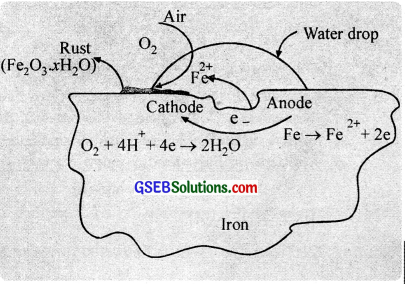The ferrous ions are further oxidized by atmospheric oxygen to form rust. Fe2O3.xH2O.
4Fe2+ + O2 + 4H2O → 2Fe2O3 + 8H+ ……………………. (4)
Fe2O3 + xH2O → Fe2O3.xH2O(s) (Rust) …………………. (5)

The two half-reactions (1) and (2) are taking place at different locations, which suggest that corrosion occurs by an electrochemical mechanism. The iron surface may be thought of as consisting of a series of tiny voltaic cells. At anodic centres, iron is oxidised to Fe2+ ions and at cathodic centres, oxygen is reduced.

Electrons are transferred through the iron, which acts as an external circuit. The electrical circuit is completed by the flow of ions through the water solution or the film covering of iron. Very pure iron does not rust easily.

But the presence of acids, salts, electrolytes, air, moisture etc accelerate the rate of rusting. Thus, (i) a perfectly dry metal surface is not attacked by oxygen (because of incomplete circuit) and (ii) corrosion occurs more readily in seawater than ¡n freshwater (seawater supplies the necessary ions for conduction).

Prevention of corrosion:

(i) By coating with a suitable material (Barrier protection):
In this method, rusting is prevented by making a barrier between the metal and the atmosphere. This is usually done by coating the surface with paints, grease, metals like Zn, Cu, Sn, Ni etc.. adherent film of iron phosphate, chemicals like bisphenol etc.

(ii) Sacrificial protection:
This method involves the coating of the surface of iron with a layer of metal which is more active than iron (e.g. Zn, Cr, Ni etc.) and this prevents the loss of electrons from iron. The active metal loses electrons in preference to iron and goes into an ionic state.

With the passage of time, the covering metal gets consumed. But as long as it ¡s present on the surface of iron, it will protect the iron from rusting and does not allow even the nearly exposed surface of iron to corrode. Zinc is commonly used to coat iron surfaces. The process of coating a thin film of zinc on iron is known as galvanisation.

Zinc ($$E_{z n^{2+} / Z n}^{0}$$ = -0.76 V) is more electropositive than iron ($$E_{Fe^{2+} / Fe}^{0}$$ = – 0.44 V), so zinc possesses greater tendency to lose electrons than iron. Thus, zinc protects iron from rusting at its own cost.

Consequently. if by reason, the protective layer of zinc is scratched, atmospheric air and moisture dissolve zinc and not iron. Thus, zinc acts as the anodic region; while iron is the cathodic region.
Zn → Zn2+ + 2e (anodic region)
H2O → H+ + OH
2H+(Fe) + 2e → H2 + Fe (cathodic region)

(iii) By cathodic protection:
In cathodic protection, iron is made as the cathode half-cell so that it will not lose electrons and dissolve. This can be achieved by attaching a more active metal such as Mg or Zn to the iron. Here the active metal acts as an anode by losing electrons and iron becomes the cathode.
(Mg → Mg2+ + 2$$\bar{e}$$)

(iv) Anti – rust solutions:
When a solution of alkaline phosphate or alkaline chromate is applied on iron objects, they form an insoluble, heat resistant iron phosphate or iron chromate coating and thus prevents rusting. Further the alkalinity of the solution removes the H+ ions which in turn decreases rusting. Such solutions are used in car radiators.

3. The OH ions supplied by the alkaline medium remove the H+ ions from the reaction,
2Fe + O2 + 4H+ → 2H2O + 2Fe2+ and retards the oxidation of Fe and inhibits the rusting of iron.Question 7.
Michael Faraday after studying the decomposition of electrolytes by electric current, put forward the laws of electrolysis?

1. State and explain Faraday’s laws of electrolysis.
2. Explain the term electrochemical equivalent.
3. Calculate the charge required to deposit 40.5 g of Al when the electrode reaction is Al3+ + 3e → Al

The amount of any substance liberated or deposited at an electrode is directly proportional to the quantity of electricity passing through the electrolyte, i.e., w ∝ Q
w = zQ
w = zlt where w – mass of substance liberated, Q – quantity of electricity passed, z – electro – chemical equivalent, I – current in amperes and t – time in seconds.

The amounts of different substances liberated by the same quantity of electricity passing through the electrolytic solution is proportional to their chemical equivalent weights.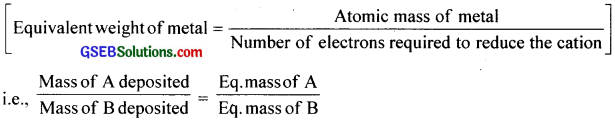The quantity of electricity required to liberate 1.008 g (I gm equivalent) of hydrogen or deposit 108 g (l gm equivalent) of silver or to liberate or deposit I gram equivalent of any substance has been found to be approximately 96500 coulombs. This quantity is called the Faraday’s constant.

On combining first and second law, it can be stated as the amount of electricity or charge required for oxidation or reduction depends on the stoichiometry of the electrode reaction. For example in the reaction Ag+(aq) + 1e → Ag(s), one mole of electron is required for the reduction of one mole of silver ion. We know that charge on one electron is equal to 1.6021 × 10-19C.

Therefore, charge on one mole of the electron is equal to 1.602 1 × 10-19C × 6.02 × 1023mol-1 = 96487 C mol-1 ~ 96500 C. This quantity of electricity is called Faraday’s constant (F).

Thus, for the electrode reaction, Mg2+ + 2e → Mg(s) requires 2 mol of electrons or 2F and for the electrons reaction, Al3+ + 3e → Al(s) requires 3 mol of electrons or 3F.

2. The electrochemical equivalent of a substance is the mass of substance liberated or deposited at an electrode by passing 1 ampere current for one second.

3. The reaction is Al3+ + 3e → Al
Charge required for depositing 1 mole Al(27 g) = 3 × 96500 C
∴ Charge required for depositing 40.5 g Al = $$\frac{3×96500×40.5}{27}$$ = 434250 C

Question 8.
What are the factors that affect the conductivity of an anionic (electrolytic) solution?
The conductivity of an anionic (electrolytic) solution depends upon the following factors.

• Temperature
• Concentration of electrolyte
• Nature of the electrolyte added
• Nature of solvent and its viscosity
• Size of the ions produced and their solvation.Question 9.
The graph showing the variation of molar conductance with concentration for acetic acid and hydrochloride acid is given below. Analyse the graph and answer the following questions?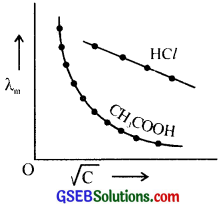1. Why does molar conductance increase with dilution?
2. Why the graphs obtained in each case has such a large difference?
3. Give the equation for the variation of molar conductivity with a concentration of strong electrolytes.
4. What is meant by molar conductance at infinite dilution? Suggest methods to find out λm for HCl and CH3OOH.

3. The molar conductance of a strong electrolyte, λm = λ°m – b$$\sqrt{C}$$, where b is a constant, C is molar concentration, λ°m molar conductance at infinite dilution.
A graph is plotted by taking $$\sqrt{C}$$ of a HCl along X-axis and λm along Y-axis. The curve when extrapolated to $$\sqrt{C}$$ = 0, the intercept gives the value of λ°m.